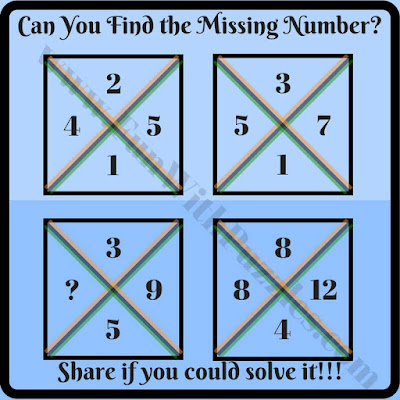## Tuesday, June 6, 2017

Learning Mathematics will be much interesting subject if Math can be tough with fun being added. Fun in Mathematics excesses can  be added with math brain teasers and riddles. One can find many different Math Brain Teasers and Riddles on the website. e.g. one can solve Circle Math puzzles in which one has to find the missing number in one of the circle out of four given circles. Similar there are Math Puzzles which are created with the shaped of square. Again in these puzzles one has to find which number will replace the question mark in one of the squares. Similar we have sequence math puzzles for kids in which one has to find the next number in the sequence. Today we are publishing cross squared math brain teasers will be add fun for school going kids while they learn basics of mathematics.
In these math brain teasers and riddles, four squares are given. Each square is divided by cross into four parts and each of the part is having one number. These numbers are related to each other by some mathematical operations. Do find out this relationship and then find the missing number in one of the square.
So are you ready to find the number which will replace the question mark? These math brain teases are easy and for school going kids. Teens and adults will find these riddle easy. Do post you answers to these puzzles in the comments.1. Can you find missing number math brain teaser riddle?2. Easy math square brain teaser riddle4. Simple math square brain teaser riddle5. What will replace question mark brain teaser riddles?

1.what is the answer for the 4th one

1.2.